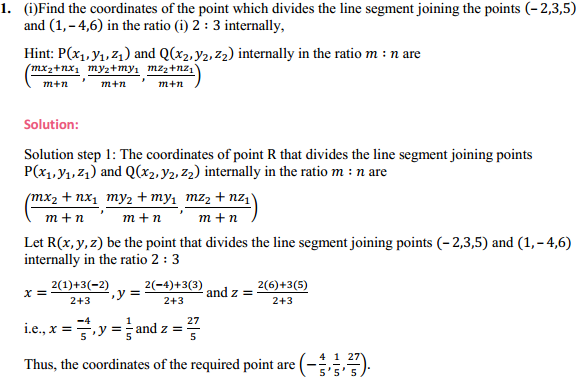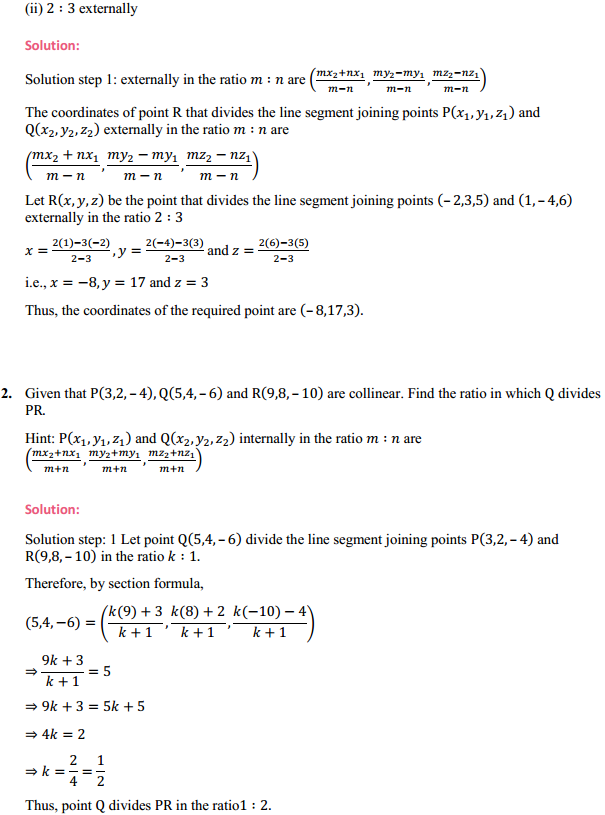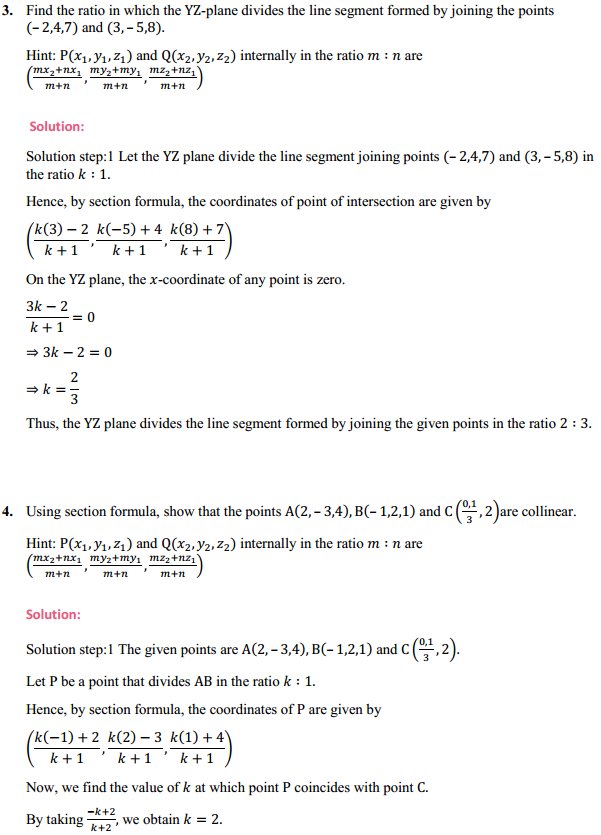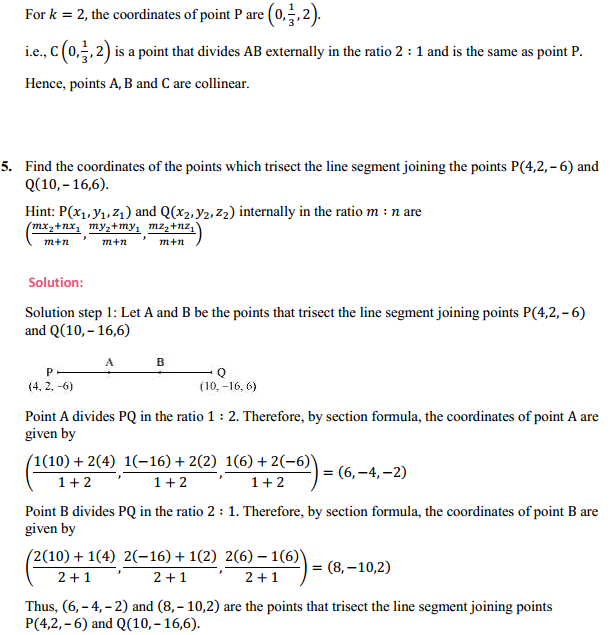# HBSE 11th Class Maths Solutions Chapter 12 Introduction to three Dimensional Geometry Ex 12.3

Haryana State Board HBSE 11th Class Maths Solutions Chapter 12 Introduction to three Dimensional Geometry Ex 12.3 Textbook Exercise Questions and Answers.

## Haryana Board 11th Class Maths Solutions Chapter 12 Introduction to three Dimensional Geometry Ex 12.3